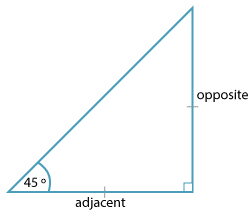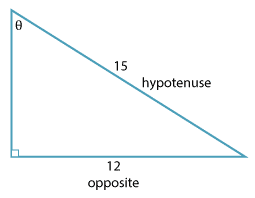### Finding angles

#### Example 2Detailed description

This is an isosceles right angled triangle and tan 45° = 1.

We can say that is the inverse tangent of 1.

We write tan$$^{−1}$$1 = 45°.

Similarly,

sin$$^{−1}$$ 0.5 = 30° as sin 30° = 0.5.

A calculator give cos 27° = 0.8910 (correct to 4 decimal places) and so:

cos$$^{−1}$$ 0.8910 $$\approx$$ 27°.

We read sin$$^{−1} \ x$$ as inverse sine of $$x$$ and cos$$^{−1}\ x$$ as inverse cosine of $$x$$.

#### Example 3Detailed description

Find the value of $$\theta$$ correct to the nearest degree.

#### Solution

This problem involves opposite and hypotenuse so use sine.

\begin{align}\text{sin} \ \theta \ &= \ \dfrac{12}{15} \\ &= \ \dfrac{4}{5} \\ \theta &= \ \text{sin}^{–1}(\dfrac{4}{5}) \\ &\approx 53.1301° \ (\text{correct to} \ 4 \ \text{decimal places}) \\ &\approx 53° \ \text{to the nearest degree}.\end{align}